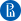up

# Discrete Math and Analyzing Social Graphs• English

course language

• 6 weeks

course duration

• от 3 до 4 часов в неделю

needed to educate

• 2 credit points

The main goal of this online course is to introduce topics in Discrete Mathematics relevant to Data Analysis.

We will start with a brief introduction to combinatorics, the branch of mathematics that studies how to count. Basics of this topic are critical for anyone working in Data Analysis or Computer Science. We will illustrate new knowledge, for example, by counting the number of features in data or by estimating the time required for a Python program to run.

Next, we will apply our knowledge in combinatorics to study basic Probability Theory. Probability is everywhere in Data Analysis and we will study it in much more details later. Our goals for probability section in this course will be to give initial flavor of this field.

Finally, we will study the combinatorial structure that is the most relevant for Data Analysis, namely graphs. Graphs can be found everywhere around us and we will provide you with numerous examples. We will mainly concentrate in this course on the graphs of social networks. We will provide you with relevant notions from the graph theory, illustrate them on the graphs of social networks and will study their basic properties. In the end of the course we will have a project related to social network graphs.

This Course is part of HSE University Master of Data Science degree program.

## Format

The course takes place on the platform of the Higher School of Economics.

The course consists of 6 weeks. Each week contains video lectures, tests and materials for independent study. At the end of the course an pratical project awaits you.

## Course program

1. Basic Combinatorics

Suppose we need to count certain objects. Can we do anything better than just list all the objects? Do we need to create a list of all our data entries to check whether we have enough data to teach our ML model? Is there a way to tell whether our algorithm will run in a reasonable time before implementing and actually running it? All these questions are addressed by a mathematical field called Combinatorics. In this module we will give an introduction to this field that will help us to answer basic versions of the above questions.

In the first week we have already considered most of the standard settings in Combinatorics, that allow us to address many counting problems. However, successful application of this knowledge on practice requires considerable experience in this kind of problems. The goal of this module is twofold. First, we study extensively more advanced combinatorial settings. We discuss in more details binomial coefficients. Also, we address one more standard setting, combinations with repetitions. The second gaol of the course is to practice counting. We will gain some experience in this by discussing various problems in Combinatorics.

3. Discrete Probability

Probability theory is a mathematical foundation of Statistics, the core of Data Science. During this week we study discrete probability, the first chapter of the probability theory, closely related to combinatorics. We discuss random experiments, their outcomes and events, introduce the notion of probability and some basic rules that follow immediately from the combinatorial results studied before. We also study simple probabilistic models like coin-tossing that will be used later.

4. Introduction to Graphs

Graphs represent objects and relations between them in a compact geometric form. Objects are represented by vertices of a graph and relations correspond to edges. Applications of graphs include geoinformational systems (vertices are cities, edges are roads), social network analysis (people and friendship relations), chemistry (graphs of molecular structure), computer network topology, and many more. During this week, we introduce basic notions of graph theory and discuss basic algorithms on graphs.

5. Basic Graph Parameters

Graph parameters, also called graph properties and graph invariants, are values (usually numerical), which are calculated for a given graph and depend only on its abstract structure (not, say, on a particular way of drawing the graph on a plane). Graph parameters are useful in data science, since they reduce a big amount of data (the graph) to a small one (the parameter), while conveying important information about the graph. We discuss some of the basic graph parameters in this module.

6. Graphs of Social Networks

In this final part of the course we discuss a Python library for working with graphs, called NetworkX. In NetworkX, one can create and modify graphs, compute graph parameters, visualize graphs, etc. We shall show how NetworkX is used to operate on graphs coming from a real-world dataset.

## Education directions

All people that work or plan to work in Data Analysis, starting from motivated high school students.### Подольский Владимир Владимирович

Доктор физико-математических наук
Position: Ведущий научный сотрудник Международной лаборатории теоретической информатики### Кузнецов Степан Львович

Кандидат физико-математических наук, Доцент
Position: Старший научный сотрудник: Факультет компьютерных наук### Щуров Илья Валерьевич

Кандидат физико-математических наук
Position: Доцент кафедры высшей математики

## Programs, which includes this course

New ProgramStart date will be announced laterНИУ ВШЭ